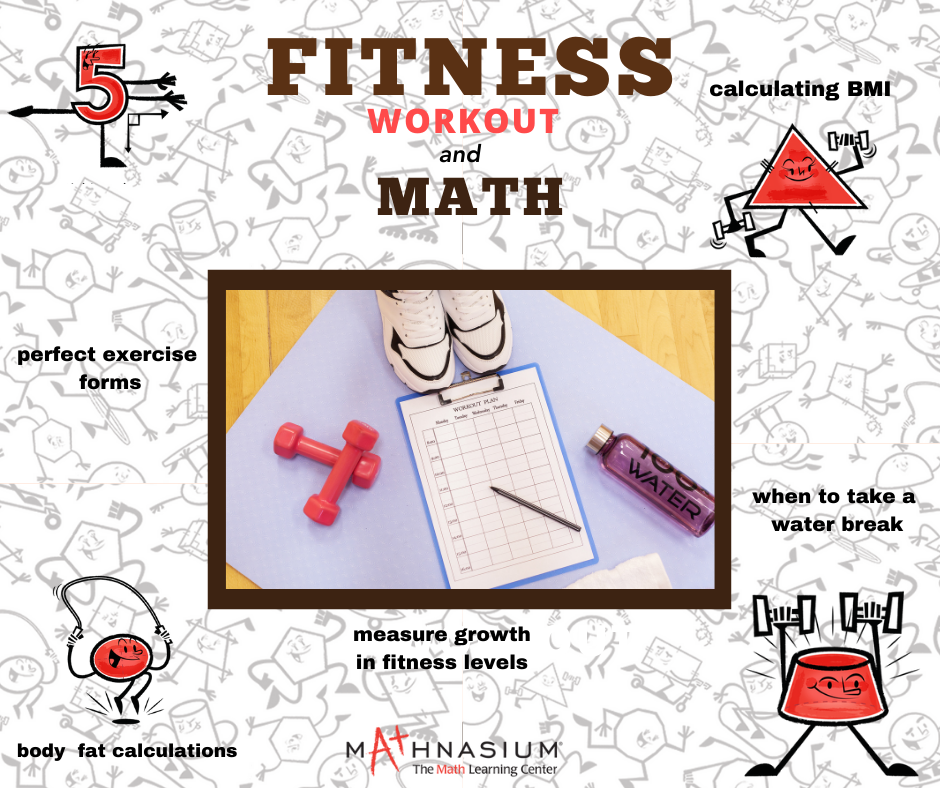877-601-6284
Get Started by Finding a Local Center

# How Is Math Used in Exercise?

May 18, 2022 | Location Bonney LakeMany aspects of our lives are dependent on math. No matter what your occupation is, there are aspects of your daily operation that require calculations. Today we will be focusing on the mathematical mechanisms within the exercise.

Do you know math can also be used in exercise and workouts?

Math can help you stay fit! While exercise isn’t typically thought of as a numerical activity, math is involved in every aspect of it. You can figure out how effective your workout is by calculating your heart rate or calories burned throughout a workout.

How is math relevant to fitness?

• Math helps us to design and maintain our exercise routines.

• Math is used to calculate short-term and long-term fitness goals.

• Math is used to measure our growth in fitness levels.

• Math can be used to perfect exercise forms.

• Math can tell us when to take a water break

Numbers & Formulas in Relation to Fitness

Heart Rate: Did you know that when we exercise, it is recommended that we make our hearts beat at a certain rate? The rate is expressed as beats per minute or BPM. However, that rate is different for all of us because it depends on our age. That rate that is specific to each of us is called our target heart rate. You use math to calculate your target heart rate for exercise. To check it put your index and middle finger on your wrist or on your neck to determine your RHR. Now multiply that amount by six to get the number of beats in 10 seconds. This rate might range anywhere from 40 to 100 beats per minute. Men tend to have an average heart rate of 70 beats per minute, while women tend to have an average heart rate of 75 beats per minute.

HRmax (Maximum Heart Rate) is the maximum heart rate a person can obtain when exercising. It is calculated as HRmax = 220 – your age

BMI (Body Mass Index): BMI is an estimate of body fat and a good gauge of your risk for diseases that can occur with more body fat. Numbers and formulas can help us calculate your BMI. You can calculate your BMI from your height and weight measurements. The higher your BMI, the higher your risk for certain diseases such as cardiovascular disease, high blood pressure, type 2 diabetes, gallstones, breathing problems, and certain types of cancers.

The standard formula for BMI is: Weight (kg) ÷ Height(m)2

Weight Conversion: wt. in-lbs ÷ 2.2 = wt. in kg

Height Conversion: ht. in inches × 0.0254 = ht. in meters

Body Fat calculations: Before beginning a fitness routine, some people choose to take measurements and calculate their body fat index. By comparing the initial numbers with the follow-up results, you can assess if you are reaching your fitness goals.

The formula for body fat calculation is

Fat Mass = % Body Fat × Body Weight

Example:

% Body Fat = 8%

Body Weight = 160 lbs

Fat Mass = 0.08 × 160 = 12.8 lbs

Lean Body Mass = Body Weight – Fat Mass

Example:

Lean Body Mass = 160 – 12.8 = 147.2 lbs

Basal Metabolic Rate: Basal Metabolic Rate (BMR) is the minimum caloric requirement needed for an individual. We calculate BMR with the help of the Harris-Benedict Equation.

Women: BMR = 655 + (4.35 × wt. in lbs.) + (4.7 × ht. in inches) – (4.7 × age)

Men: BMR = 66 + (6.23 × wt. in lbs.) + (12.7 × ht. in inches) – ( 6.8 × age)

Use Math to Maintain Your Diet

Mathematical algorithms can also be used to determine calorie intake from nutritional labels. As a result, you get a better understanding of how carbohydrates, protein, and fat are converted into calories and how this affects portion size.

For example, suppose you order a medium pizza with eight slices and eat two of them. What fraction of the pizza did you eat? You’ve eaten 2/8 or ¼ (one quarter) of the pizza. Now, if you want to find out how many calories are in that 1/4 of a serving, convert the fraction into a decimal, then multiply it by the number of calories in the serving. Suppose each serving has 450 calories, Multiply 450 X 0.25 = 112.5 calories. So, two slices of pizza that you ate had 112.5 calories.

Math is a useful tool to optimize our diet and exercise to live healthier life. The more mathematical we are in our approach, the more grounded our thoughts are!

##### Locations near
Could not find Center, try again